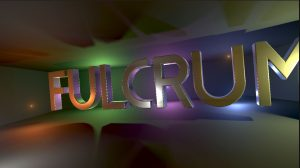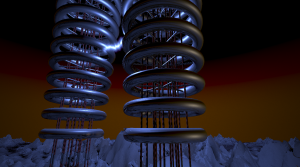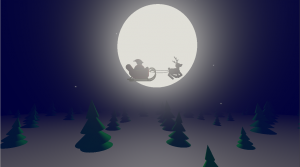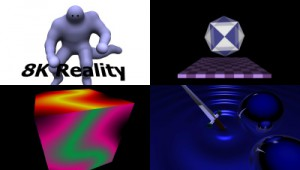# Category: News

This is the exhaustively documented shader code of the Jumpgate 10.24 1 kilobyte intro.

```/*
Jumpgate 10.24 by Seven/Fulcrum
-------------------------------
This is the documented sourcecode for the shader of my 1 kilobyte intro for Assembly
2020. Apart from the whitespace for readability, it's identical to the shader used
in the final 720p version.

I'm sharing this so can you learn from it (especially from my mistakes), so don't copy
it wholesale, don't use it for work purposes :D and of course I have no
resposibility if anything bad happens. It's a 1K, not a shining example of
safety and clarity!

*/

// m is the time. A simple frame counter is passed via gl_color, I rely on automatic
// truncation to get the x component. There is a scaling factor to speed up or slow down the entire
// intro. The offset .65 ensures the ship reaches the jumpgate at time 0. We're going to see this number
// a lot. f is used for various things, d is the random seed for the ship generation routine.
float m=10.5*gl_Color-.65,f,d;

// standard 2D rotation routine, rotates a vec2 m radians. I think H4rdy/Lemon shared this variant first.
// Named s for StandardRotationFunction
vec2 s(vec2 y,float m)
{
return y*cos(m)+vec2(y.y,-y.x)*sin(m);
}

// NSA-approved random function. We're just trying to get some variation and be very compressible,
// not to be cryptographically secure :) The seed is increased locally, and some simple math is done.
// Note the use of frac() instead of fract(), which is an HLSL function that the nvidia drivers accept
// with a warning, but AMD errors on it. I normally don't stoop to exploiting brand-dependent differences,
// but since I already made a mistake that made the intro nvidia-only, I might as wel save some bytes...
// The .65 could have been any number, but I reused a number for maximum compression.
// Named s for SomewhatRandom
float s()
{
return d++,frac(d*.65*frac(d*.65));
}

// The heart of the intro: the Signed Distance Function (SDF) for the procedurally generated ships/jumpgate.
// y is the 3D point for which to evaluate the SDF, m is the random seed for this specific spaceship.
// Named s for ShipSDF
float s(vec3 y,float m)
{
// Initialize the distance as "far away". Any big number is OK so try to use one that's needed elsewhere.
float f = 154;
// initialize the random seed before calling s() with abandon.
d=m;

// The basic shape of the ship is a randomly-oriented plane, shifted from the origin, and then mirrored
// around 2 axis. This typically gives you an octahedron (a pyramid on top of an upside-down pyramid).
// Then I uses IQ's elongation function, which add bevels to the octahedron. But it's simplified which
// causes the elongation to only happen on the positive side of each axis, so the shape is not centered
// on the origin anymore.
// We create 4 of those, but each time, they're 4 times as many (due to mirroring), a bit smaller and
// shifted more to the rear. So we get one big octahedron (body/cockpit), with 4 smaller ones a bit more
// to the back (wings?), with 16 even smaller(engines?), and 64 smallest (random parts) at the end.
// Depending on the random function, some of these might not be visible. We also rotate the octahedra to
// generate wings etc.

// 4 levels of parts
for(float d=0;d<4;d++)
{
// elongate a random amount.
y-=min(max(y,0),s());
// mirror X and Y axis.
y.xy=abs(y.xy);

// Combine the SDFs of each part with the min operator
f=min(f,
pow(.7,d) // Scale the result up. This SHOULD be the same as 1/scalefactor (which is 1.6), but you
// can abuse this as a kind of safety factor (to hide artifacts of other bugs) or as an
// overstepping factor (to speed up marching, if other functions are too conservative).
// So you can cheat a bit and re-use some number (like .65), and then suffer from it when
// your intro contains artifacts and you can't fix it without breaking the filesize...
*(dot(abs(y),	// use the dot function as a SDF for a plane oriented by it's normal.
normalize(vec3(s(),s(),s()*.2) // random normal, tweaked to get octahedra stretched along Z-axis
// ALSO UNDEFINED BEHAVIOR THAT BREAKS ON AMD!
+.01) // to prevent too many very thin needle shapes, add a constant before normalizing.
-s())); // this is the thickness of the plane, randomly picked.

// So, did you figure out the undefined behavior? The order of evaluation of paramaters is not defined in GLSL.
// So if your random function generates 0.3, 1, and 0.5, nvidia will give you vec3(.3, 1, .5), but AMD might
// give you vec3(.5,1, .3)... In the safe versions, this is fixed with extra variables: a=s(), b=s(), c=s(),
// ... vec3(a,b,c); , But I didn't had room for that in the compo version :( Sorry, AMD fans.

// Scale the octahedra down, and shift them a random amount, but mostly to the back of the plane.
// Also undefined behavior again.
y=y*1.6-vec3(s(),s(),s()*-3);
//Finally, rotate the next part a random amount around the z-axis
y.xy=s(y.xy,s());
}
return f;
}

// This is the SDF for the entire fleet and the jumpgate.
// Named s for SceneSDF
float s(vec3 y)
{
// The jumpgate is just the back end of a carefully-chosen ship, scaled up, and combined with a sphere.
// Remember that the elongation operation was not symmetrical anymore? That means we have to shift the
// gate back a bit (.15) so it matches the sphere.
float f=min(length(y)-14, // the sphere
s(y/9+.15,8.8)*9); // jumpgate, seed 8.8 (I tried hundreds of combinations), scaled by 9.

// This generates the fleet. For maximum compression (have you heard that before?), I use the same
// loop variable and iteration count as the ship SDF. So we have 4 different ship types. Of course, the
// higher the amount of ship types, the longer you have to look for a seed that generates decent ones
// for ALL types. So this is another giant timesink, and every time you tweak a constant in the ShipSDF,
// you have to start over :(
// The fleet is build up the same as each individual ship: 4 layers, each layer scaled down and mirrored.
// So that should give us 1 big ship, 4 medium, 16 small, 64 tiny. There is no rotation, because then it
// looked like a traffic accident instead of an organised fleet. But the resulting pyramid (big ship at
// top, smaller ones below) looked far too regular, with perfectly-alligned layers of ships, so I
for(float d=0;d<4;d++)
{
// Offset the ships from each other. This would make a line from big ship to small ship.
y-=vec3(18,9,50);

// mirror the X and Y direction, causes more smaller ships.
y.xy=abs(y.xy);

// Here's the extra trick: shift mirrored space back! This cause another 4 extra ships
// to appear in front of each original. Depending on the camera angle, it also wreaks havoc with your
// SDF accuracy, so you better like futzing around with parameters to hide marching artifacts :(
if(y.z>13)
y-=vec3(8,6,26);

// Combine the SDFs of each ship with the min operator
f=min(f,
pow(m+1.1,d) // scaling correction. Giant hack ahoy: the smallest ships had bad overstepping artifacts
// which were fixed by decreasing this, but that caused the jumpgate to disappear behind the
// big ships. Since they are at different times in the intro, I use the time m (which starts at
// -.65) to adjust the scaling differently during the intro.
*s(y,d+.31)); // Ship SDF, with seed .31 + the layer index.

// Scale the fleet (same factor as ship parts, FOR GREAT COMPRESSION!!). Shifting the ships around
// is kind of useless since each layer is shifted the same amount, but it improved compression at some point.
y=y*1.6-vec3(s(),s(),s() -3);
}
return f;
}

void main()
{

vec3 v,		// the current point we're evaluation on the camera ray from this pixel.
z=normalize(-vec3(1,.55,2)+.0015*gl_FragCoord); // the raymarch direction, for a 720p screen.
// Note abuse of truncation of gl_FragCoord again.

// When the camera reaches the gate, we want it to enter straight ahead. So get a time value that becomes 0 near the gate.
f=min(m+.1,0);
// rotate the camera left/right (one sweep) and up/down (sine wave).
// The f*f*(2-f) is IQ's near-identity function to smoothly halt the camera motion.
z.yz=s(z.yz,f*f*(f-2)*sin(m*-3.5));
z.xz=s(z.xz,f*f*(f-2)*4);

// The actual raymarching. No early exit at all. The camera position is defined in the parameter.
// f is the total distance travelled. It starts as the time value instead of 0,but that's OK as
// we're not doing close-ups
// z is the direction, v is the current position.
for(float d=0;d<104;d++)
f+=s(v=vec3(0,0,-m*274)+z*f);

vec3 y=-vec3(1,0,.2), // the sun direction, slightly higher than 1 for dramatic lighting and better compression
x=normalize(vec3(s(v+vec3(.01,0,0)),	// the normal, calculated by the usual 3 evaluation method
s(v+vec3(0,.01,0)), // plus the assumption we are perfectly on the surface.
s(v+vec3(0,0,.01)))),
r=pow(max(0,dot(z,reflect(y,x))),104)  // specular lighting
+vec3(.31,.4,.5)*(max(0,dot(y,x))	  // diffuse lighting, on blue-ish material
+.5);   // ambient lighting

z=s(v)<.01?reflect(z,x):z; // if we've hit something, reflect the ray direction using the normal.

// The first of 3 very similar fractals. This is based on Knighty's Cosmos fractal (on Shadertoy.com)
// but simplified because I just need a static background. The main concern is to get stars that are
// not too big, but not tiny either because those flicker like hell in motion.
for(float d=0;d<15;d++) // 15 layers
{
y=vec3(.65,.2,d*.004)-z*d*.004; // startpoint depends on ray direction and carefully recycled constants.
for(float d=0;d<15;d++) // 15 iterations
f=dot(y,y),y=abs(y)/f-.65; // get square of distance (dot), mirror (abs) and shift (-.65)
x-=f*y; // color the distance f with the endposition y, which gives pretty coherent colors.
}

// The ships red coloring. Only show it if we didn't hit the gate sphere.
if(length(v)>14.1)
{
y=v-84; // scaling factor to get somewhat interesting patterns on most ships (not full red/blank)
for(float d=0;d<15;d++) // 15 iterations
f=length(y),y=abs(y)/f-.65; // get distance (length), mirror (abs) and shift (-.65)

// blank the green and blue components. The treshold makes the red or blank parts dominate.
if(f<.3)
r.yz=0; // add this to the material color, not the background
}
// The jumpgate sphere fractal. Only show if we hit the sphere.
if(length(v)<14.1)
for(float d=0;d<15;d++) // 15 layers
{
y=vec3(0,0,-m*30-1)-z; // the startpoint is chosen so the inversion happens at teh right time.
for(float d=0;d<15;d++) // 15 iterations
f=length(y),y=abs(y)/f-.65; // This fractal really, really depends on the .65 value.
x+=f*154; // add the fractal to the background, with appropriate brightness
}

// depending on wether we hit something, show the background, or the material with reflected background.
gl_FragColor.xyz=s(v)<.01?r+x*.0004:x*.004;

}
```

## MAGIIt took us 7 years to release a new demo, and one month later we have another ready :) Made in approximately 1 week time to support the MAGFest 2019 demoparty, it took 1st place in the combined intro/demo competition. It’s a bit short, but we’re happy we made it.

Get the executable here: MAGI by Fulcrum

If your videocard is too slow, watch it on Youtube: MAGI by Fulcrum

## Arctic Voltage 4KHere is our contribution to the 4K intro competition at Under Construction 2018.

Get the executable here: Arctic Voltage by Fulcrum

## Shoot The Core 1KThis is our contribution to the Assembly 2017 1K intro compo. You can download the executable here:
Shoot The Core 1k By Fulcrum

## Naughty Or Nice 4KThis is our yearly Christmas contribution to the Under Construction 2014 party.

## 4K Compo Stocking FillerHere’s a little party-coded compofiller 4K for the Under Construction 2015 demoparty. I was aiming for a more realistic Christmas tree, but the result has tons of raymarch glitches and runs very slow… Still, the audience appreciated it for what it was. Huge thanks to Skyrunner/Brain Control for helping out with the music in a hurry.

Get the executable here: 4K Compo Stocking Filler by Fulcrum

## 8k Reality – The Soundtrack

Along with Seven’s write up on coding an 8k, I did a write up on the 8k Reality soundtrack. Read it here.

## 8K Reality (final)This is our contribution to the Revision 2015 8K intro competition, where it placed 2nd.
Its a remake of Second Reality, the famous demo by Future Crew, in 8192 bytes . (No, 8K has nothing to do with the resolution)

You can download the final version with more transitions but no voices, including low-res version and 12K versions with higher-quality soundtrack: 8K Reality by Fulcrum (final)

Feedback welcome on Pouet: http://www.pouet.net/prod.php?which=65412

And now for the making-of, which is an expanded and illustrated version of the readme:

## Regression (soundtrack)

The final mix of the soundtrack to the Regression 8k intro.

Regression 8k Soundtrack

## Regression 8k takes 2nd place at Revision 2014

Check it out here

Results here